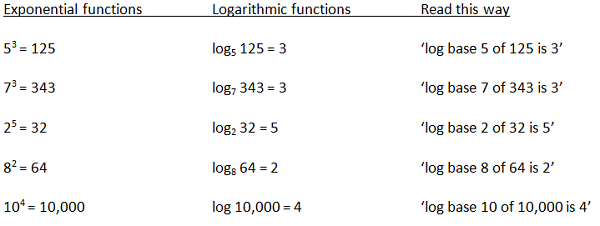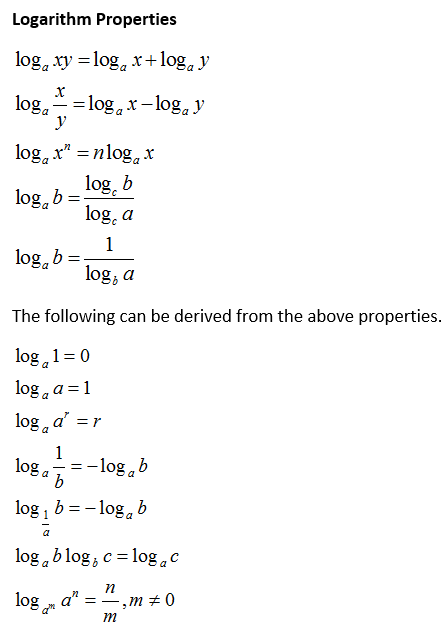### Logarithm

Inverse function to exponentiation is called logarithm. the logarithm of a given number is the exponent to which another fixed number, the base, must be raised, to produce that number.Let the given no.is ‘x’,the base is ‘b’, must be raised to produce that no.’x’.

The defining relation between exponentiation and logarithm is

logb(x)=y

Where b and x any two positive real numbers and  b ≠1

Exactly if =x

For example:

log2(64)=6, as 26 = 6

The logarithm to base 10 (that is b = 10) is called the common logarithm and has many applications in science and engineering. The natural logarithm has the number e (that is b ≈ 2.718) as its base; its use is widespread in mathematics and physics, because of its simpler integral and derivative. The binary logarithm uses base 2 (that is b = 2) and is commonly used in computer science.

the logarithm of a product is the sum of the logarithms of the factors:

logb(xy) = logb(x) + logb(y)

Where b,y and x, any two positive real numbers and b ≠1

{\displaystyle \log _{b}(xy)=\log _{b}x+\log _{b}y,\,}Logarithmic scales reduce wide-ranging quantities to tiny scopes.

For example,

The decibel (dB) is a unit used to mostly for signal power and  amplitude in terms of logarithm.

In chemistry,pH is a logarithmic measure for the acidity of an aqueous solution.

Logarithms are commonplace in scientific formulae, and in measurements of the complexity of algorithms and of geometric objects called fractals.

Relation between exponential and logarithm​​ Some Logarithm Properties: